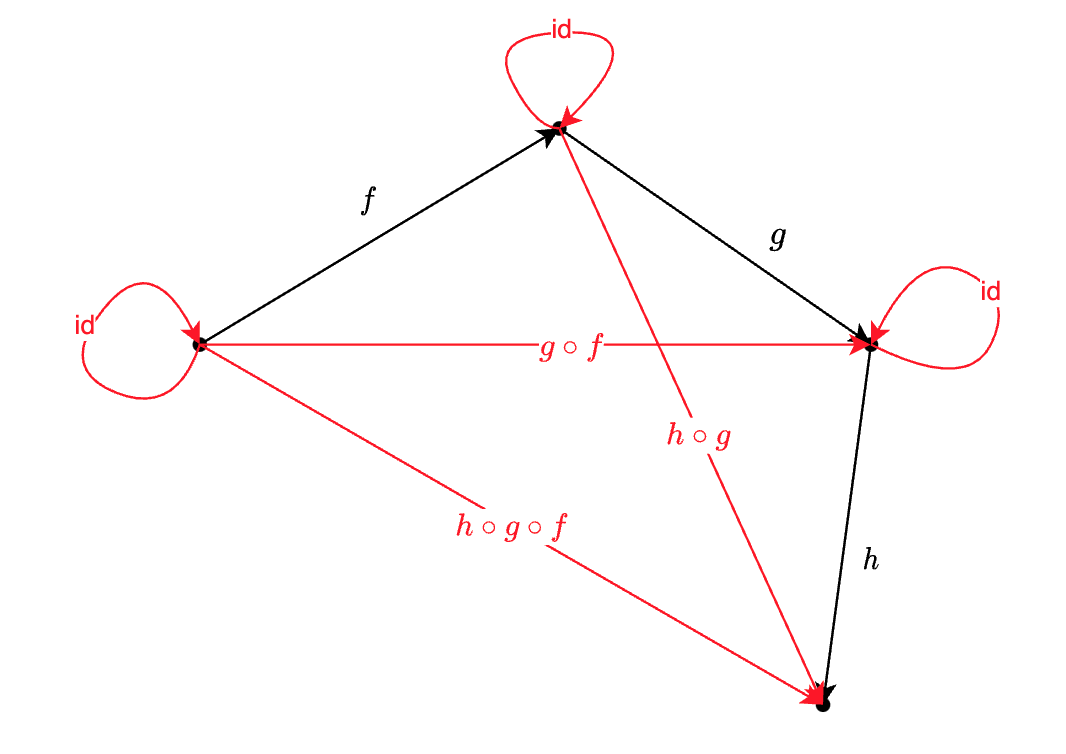# oldhu's

### Category是什么

Category 中包含两个类别的内容：objectmorphism

• objectcategory 中的点
• 一个点向另一个点的变换，称为 morphism (态射)

Category 必须满足以下3点要求(才能被称为一个 Category)：

1. $f: a \rightarrow b$ 和 $g : b \rightarrow c$ 可以组合(compose) 成 $g \circ f$ (读作g after f) ，并且：$g \circ f = a \rightarrow c$
2. 如果存在 $f: a \rightarrow b$ 和 $g : b \rightarrow c$ 和 $h : c \rightarrow d$ ，则 $h \circ ( g \circ f )$ 与 $( h \circ g ) \circ f$ 等价。最终的结果都是 $a \rightarrow d$。
3. 存在$id_x : x \rightarrow x$，使得 $f \circ id_a = id_b \circ f = f$Category 中的 object ，不承载任何信息，承载信息的(我们重点关注的)是点和点之间的关系(morphism)

### Category与编程中的概念如何对应

Category 大致可以与编程中的一个类型对应起来。比如如果将整数作为一个 Category，那么object就是所有的整数的值。morphism就是整数与整数之间的变换。